# OliNo

Renewable Energy

## Line Lite International BV LedTL 1200mm WWLine Lite International BV offers an LED-TL with a lot of leds. This 1200 mm warm white version is tested here.

See this overview for a comparison with other light bulbs.

### Summary measurement data

parameter meas. result remark
Color temperature 3093 K Warm white
Luminous intensity Iv 665 Cd
Beam angle 69 deg
Power P 14.3 W
Power Factor 0.50 For every 1 kWh net power consumed, there has been 1.7 kVAhr for reactive power.
Luminous flux 932 lm
Luminous efficacy 65 lm/W
CRI_Ra 67 Color Rendering Index.
Coordinates chromaticity diagram x=0.4375 and y=0.4168
Fitting FL-tube
L x D external dimensions 1200 x 30 mm External dimensions of the light bulb.
L x W luminous area 1090 x 26 mm Dimensions of the luminous area (used in Eulumdat file). This is the surface area of the plate on which the leds are mounted.
General remarks The ambient temperature during the whole set of measurements was 22.5-26 deg C.

Warm up effect: illuminance decreases less than 10 % during warm up of the tube.

Voltage dependency: little (<5%)no dependency. At voltages below 210 V the illuminance drops quickly.

The Eulumdat file can be found via this link.

Measurement report (PDF)### Eulumdat light diagram

An interesting graph is the light diagram, indicating the intensity in the C0-C180 and the C90-C270 plane. This light diagram below comes from the program Qlumedit, that extracts these diagrams from an Eulumdat file.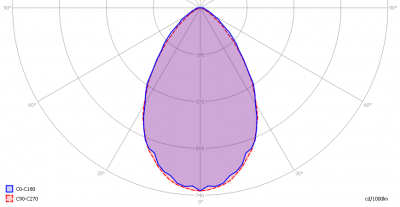The light diagram giving the radiation pattern.

It indicates the luminous intensity around the light bulb. This light diagram is symmetrical to plane C0-C180 (perpendicular to length side of the lamp) and symmetrical to plane C90-C270 (alongside the length of the lamp).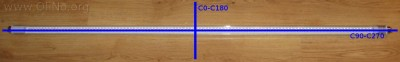The planes indicated.

Both the C0-C180 and C90-C270 planes look like a cosine shape pattern, since it represents the ends of the lamp.

The unit is Cd/1000lm, meaning the intensity in Cd assuming there would be 1000 lumen in the measured light bulb. This enables comparing different types of light bulbs.

### Illuminance Ev at 1 m distance, or luminous intensity Iv

Herewith the plot of the averaged luminous intensity Iv as a function of the inclination angle with the light bulb.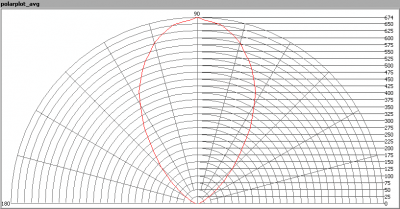The radiation pattern of the light bulb.

This radiation pattern is the average radiation, so per inclination angle the intensity results of all turn angles are averaged. This graph gives less information than does the Eulumdat light diagram graph. The results of averaging are needed to compute the lumen output.

This graph is not normalized to 1000 lm, but instead the intensity is directly given for this lamp.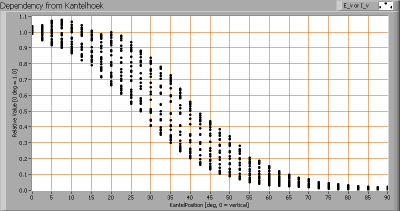Intensity data of every measured turn angle at each inclination angle.

This plot shows per inclination angle the intensity measurement results for each turn angle at that inclination angle. So at 45 degrees inclination angle, all the measurement results of illuminance for all measured turn angles are in the range of 13-42 % of the illuminance value at 0 degrees inclination angle.

When using the average values per inclination angle, the beam angle can be computed, being 69 degrees.

### Luminous flux

With the averaged illuminance data at 1 m distance, taken from the graph showing the averaged radiation pattern, it is possible to compute the luminous flux.

The result of this computation for this light spot is a luminous flux of 932 lm.

### Luminous efficacy

The luminous flux being 932 lm, and the power of the lightbulb being 14.3 W, yields a luminous efficacy of 65 lm/W.

A power factor of 0.50 means that for every 1 kWh net power consumed, a reactive component of 1.7 kVAr was needed.

 Light bulb voltage 230 V Light bulb current 125 mA Power P 14.3 W Apparent power S 28.7 VA Power factor 0.50

### Color temperature and Spectral power distributionThe spectral power distribution of this light bulb.

The measured color temperature is about 3100 K which is warm white.

This color temperature is measured straight underneath the light bulb. Below a graph showing the color temperature for different inclination angles.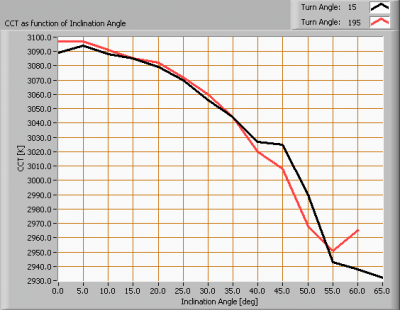Color temperature as a function of inclination angle.

The maximum value of inclination angle is 60 degrees. Beyond that value there is virtually no intensity anymore. The color temperature decreases 3 % with increasing inclination angle.

### Chromaticity diagram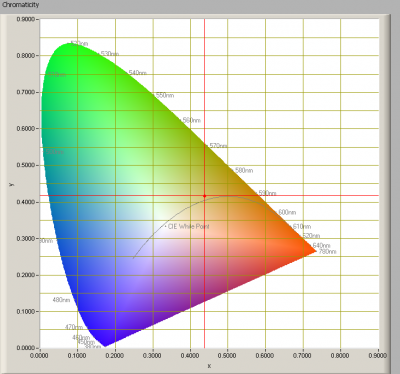The chromaticity space and the position of the lamp’s color coordinates in it.

The light coming from this lamp is at a distance from the Planckian Locus (the black path in the graph).

Its coordinates are x=0.4375 and y=0.4168.

### Color Rendering Index (CRI) or also Ra

Herewith the image showing the CRI as well as how well different colors are represented (rendered). The higher the number, the better the resemblance with the color when a black body radiator would have been used (the sun, or an incandescent lamp).

Each color has an index Rx, and the first 8 indexes (R1 .. R8) are averaged to compute the Ra which is equivalent to the CRI.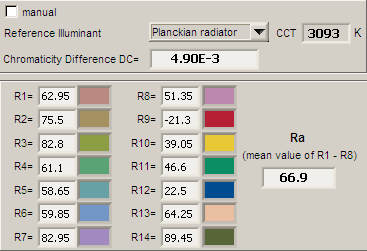CRI of the light of this lightbulb.

The value of 67 is lower than 80 which is considered a minimum value for indoor usage.

Note: the chromaticity difference is 0.0049 indicates the distance to the Planckian Locus. Its value is lower than 0.0054, which means that the calculated CRI result is meaningful.

### Voltage dependency

The dependency of a number of lamp parameters on the lamp voltage is determined. For this, the lamp voltage has been varied and its effect on the following lamp parameters measured: illuminance E_v [lx], color temperature CT or correlated color temperature CCT [K], the lamppower P [W] and the luminous efficacy [lm/W].Lamp voltage dependencies of certain light bulb parameters, where the value at 230 V is taken as 100 %.

The consumed power and the illuminance are little dependent from lamp voltage variation (less than 5 %). The illuminance and consumed power drop quickly when decreasing voltage below 210 V.

When the lamp voltage varies between 230 + and – 5 V, the illuminance varies less than 0.3 %. So sudden voltage variations in the lamp voltage will not be visible.

### Warm up effects

After switch on of a cold lamp, the effect of heating up of the lamp is measured on illuminance E_v [lx], color temperature CT or correlated color temperature CCT [K], the lamppower P [W] and the luminous efficacy [lm/W].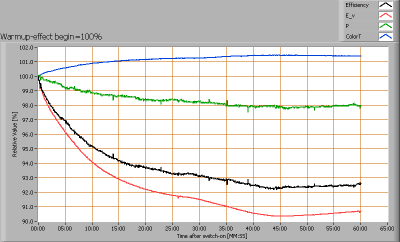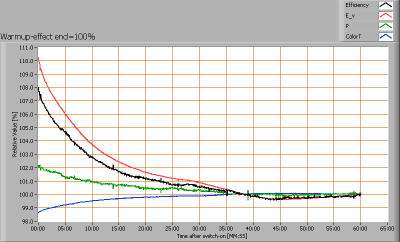Effect of warming up on different light bulb parameters. At top the 100 % level is put at begin, and at bottom at the end.

The illuminance decreases 10 % during its warmup time of 30 minutes.